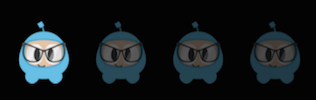## Sprite Manipulation

After creating a `Sprite` you will have access to a variety of properties it has that can be manipulated.

Given:

``````auto mySprite = Sprite::create("mysprite.png");
``````### Anchor Point and Position

Anchor Point is a point that you set as a way to specify what part of the `Sprite` will be used when setting its position. Anchor Point affects only properties that can be transformed. This includes scale, rotation, skew. This excludes color and opacity. The anchor point uses a bottom left coordinate system. This means that when specifying X and Y coordinate values you need to make sure to start at the bottom left hand corner to do your calculations. By default, all `Node` objects have a default anchor point of is (0.5, 0.5).

Setting the anchor point is easy:

``````// DEFAULT anchor point for all Sprites
mySprite->setAnchorPoint(0.5, 0.5);

// bottom left
mySprite->setAnchorPoint(0, 0);

// top left
mySprite->setAnchorPoint(0, 1);

// bottom right
mySprite->setAnchorPoint(1, 0);

// top right
mySprite->setAnchorPoint(1, 1);
``````

To represent this visually: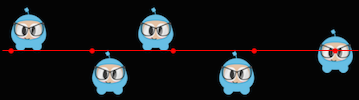### Sprite properties effected by anchor point

Using anchor point effects only properties that can be transformed. This includes scale, rotation, skew.

#### Position

A sprite's position is affected by its anchor point as it is this point that is used as a starting point for positioning. Let's visually look at how this happens. Notice the colored line and where the sprite's position is in relation to it. Notice, as we change the anchor point values, the sprite's position changes. It is important to note that all it took was changing the anchor point value. We did not use a `setPosition()` statement to achieve this: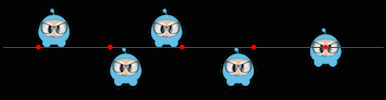There are more ways to set position than just anchor point. `Sprite` objects can also be set using the `setPosition()` method.

``````// position a sprite to a specific position of x = 100, y = 200.
mySprite->setPosition(Vec2(100, 200));
``````

#### Rotation

Changes the sprite's rotation, by a positive or negative number of degrees. A positive value rotates the `Sprite` object clockwise, while a negative value rotates the `Sprite` object counter-clockwise. The default value is 0.

``````// rotate sprite by +20 degrees
mySprite->setRotation(20.0f);

// rotate sprite by -20 degrees
mySprite->setRotation(-20.0f);

// rotate sprite by +60 degrees
mySprite->setRotation(60.0f);

// rotate sprite by -60 degrees
mySprite->setRotation(-60.0f);
``````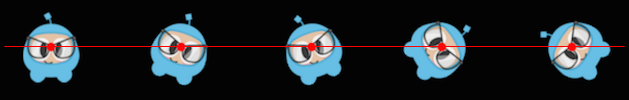#### Scale

Changes the sprite's scale, either by x, y or uniformly for both x and y. The default value is 1.0 for both x and y.

``````// increases X and Y size by 2.0 uniformly
mySprite->setScale(2.0);

// increases just X scale by 2.0
mySprite->setScaleX(2.0);

// increases just Y scale by 2.0
mySprite->setScaleY(2.0);
``````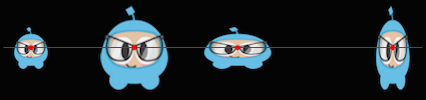#### Skew

Changes the sprite's skew, either by x, y or uniformly for both x and y. The default value is 0,0 for both x and y.

``````// adjusts the X skew by 20.0
mySprite->setSkewX(20.0f);

// adjusts the Y skew by 20.0
mySprite->setSkewY(20.0f);
``````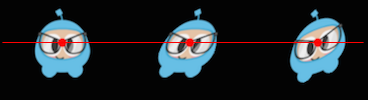### Sprite properties not affected by anchor point

There are a few properties of `Sprite` objects that are not affected by anchor point. Why? Because they only change superficial qualities like color and opacity.

#### Color

Changes the sprite's color. This is done by passing in a `Color3B` object. `Color3B` objects are RGB values. We haven't encountered `Color3B` yet but it is simply an object that defines an RGB color. An RGB color is a 3 byte value from 0 - 255. Cocos2d-x also provides pre-defined colors that you can pick from. Using these will be a bit faster since they are pre-defined. A few examples: `Color3B::White` and `Color3B::Red`.

``````// set the color by passing in a pre-defined Color3B object.
mySprite->setColor(Color3B::WHITE);

// Set the color by passing in a Color3B object.
mySprite->setColor(Color3B(255, 255, 255)); // Same as Color3B::WHITE
``````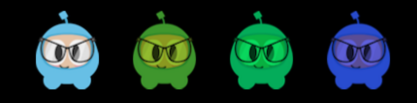#### Opacity

Changes the sprite's opacity by the specified value. An opaque object is not transparent at all. This property expects a value from 0 to 255, where 255 means fully opaque and 0 means fully transparent. Think: zero opacity means invisible, and you'll always understand how this works. The default value is 255 (fully opaque).

``````// Set the opacity to 30, which makes this sprite 11.7% opaque.
// (30 divided by 256 equals 0.1171875...)
mySprite->setOpacity(30);
``````